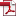## Equally Spaced 기약다항식 기반의 효율적인 이진체 비트-병렬 곱셈기

Vol. 18, No. 2, pp. 3-10, 4월. 200810.13089/JKIISC.2008.18.2.3, Full Text:Keywords: Bit-Parallel Multiplier, redundant representation, Left-to-Right expoentiation, Equally spaced irreducible polynomial
Abstract

유한체 \$GF(2^m)\$의 원소를 표현하기 위한 기저선택은 곱셈기의 효율성에 영향을 미친다. 이중에서 여분표현을 이용한 곱셈기는 모듈러 감산을 빠르게 구성할 수 있는 특징을 이용하여 시간-공간의 trade-off를 효율적으로 제공한다. 따라서 여분표현을 이용한 기존의 곱셈기는 다른 기저로 표현한 곱셈기보다 시간 복잡도 상의 효율성을 제공하나 공간 복잡도가 많이 늘어나는 단점을 가진다. 본 논문에서는 다항식 지수승 연산이 많이 사용된다는 것을 감안해 Left-to-Right 형태의 지수승 환경에 적합한 시간-공간 복잡도 상의 효율성을 가지는 새로운 비트-병렬 곱셈기를 제안한다. 제안하는 곱셈기는 \$T_A+({\lceil}{\log}_2m{\rceil})T_x\$ 시간 복잡도와 (2m-1)(m+s) 공간 복잡도를 요구하며 ESP(Equally Spaced Polynomial) 기약다항식 기반의 기존 여분표현 곱셈기와 비교해 공간 복잡도는 \$2(ms+s^2)\$ 감소하며, 시간복잡도는 \$T_A+({\lceil}{\log}_2(m+s){\rceil})T_x\$에서 \$T_A+({\lceil}{\log}_2m{\rceil})T_x\$로 감소된다. (\$T_A\$:2개의 입력에 1개의 출력인 AND 게이트 시간, \$T_x\$:2개의 입력에 1개의 출력인 XOR 게이트 시간이며 m:ESP기약 다항식 차수, s: ESP기약 다항식의 각항의 차수 간격)

Statistics